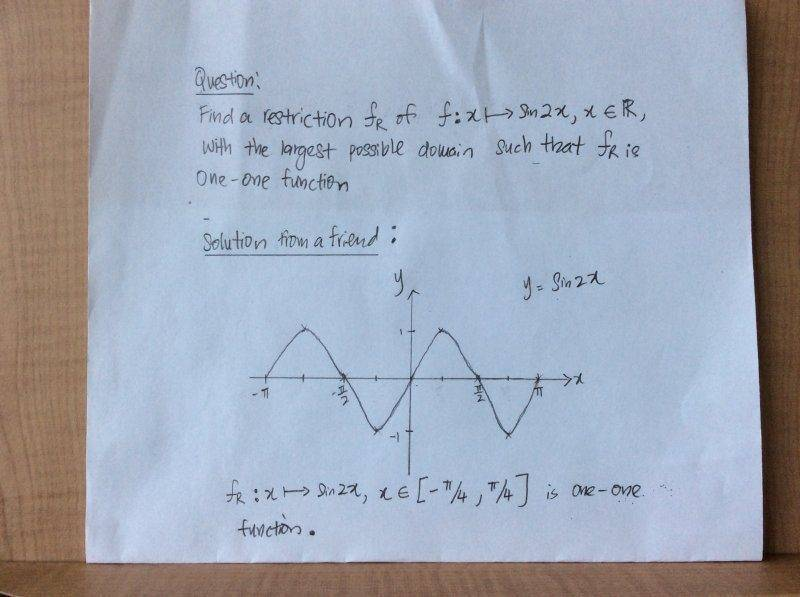# One-one function

## Homework Statement

Dear Mentors and PF helpers,

[/B]## Homework Equations

My understanding of a one-one function is that if a horizontal line where to intersect the curve, there should be only 1 intersection.[/B]

## The Attempt at a Solution

1) My questions is what does "the largest possible" domain means?

Does it mean to find the widest interval between the lower limit and the higher limit? Or does it mean that the upper limit should be as great as possible?

Mark44
Mentor

## Homework Statement

Dear Mentors and PF helpers,

[/B]
View attachment 77164

## Homework Equations

My understanding of a one-one function is that if a horizontal line where to intersect the curve, there should be only 1 intersection.[/B]

## The Attempt at a Solution

1) My questions is what does "the largest possible" domain means?

Does it mean to find the widest interval between the lower limit and the higher limit? Or does it mean that the upper limit should be as great as possible?
No, it means the largest interval on the horizontal axis for which the function is one-to-one.

Dear Sirs,

Than can the answer be [ pi/4, 3pi/2].

Thank you

Mark44
Mentor
Dear Sirs,

Than can the answer be [ pi/4, 3pi/2].
No, that's too large an interval.

Dear Sirs,

Why it is too large an interval? That section that I mentioned is also within the definition of a one one function. Why it is that we have to specifically choose [-pi/4,pi/4], there are many sections to choose as well.

Mark44
Mentor
Dear Sirs,

Why it is too large an interval?
Because your function is not one-to-one on that interval (i.e., on ##[\pi/4, 3\pi/2]##. Did you mean ##[\pi/4, 3\pi/4]## instead?
LiHJ said:
That section that I mentioned is also within the definition of a one one function. Why it is that we have to specifically choose [-pi/4,pi/4], there are many sections to choose as well.
Yes, any interval of the right length will do. What you want is an interval on which the function is either increasing on the entire interval or decreasing on the entire interval.

Dear Sirs,

Sorry I typed wrongly, ya i mean [ pi/4, 3pi/4 ]. So my answer should be acceptable as well?

Mark44
Mentor
Dear Sirs,

Sorry I typed wrongly, ya i mean [ pi/4, 3pi/4 ]. So my answer should be acceptable as well?
I'm not sure. The question was "what is the largest possible domain?" so I think they are looking for an interval length rather than an interval. The length of the largest possible domain is ##\pi/2##.

Thank you very much;)